×

### Html & Html5

Introduction to Html

Html5 Grid

Html5 Graphics

Html5 Media

### Python Lambda

A lambda function is a small anonymous function that defines without a name. Python anonymous function are defined using the lambda keyword.

Syntax :

Lambda argument:expression
let's have an example :-

double=lambda x:x*5

print(double(5))

### Output of this Program

Here in above program we just create lambda function which will give us the multiply of the argument which we have passed .
Let’s see what happens :-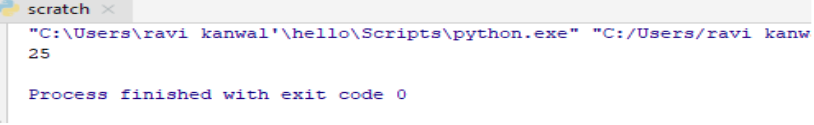So here we get our desired value .

We can also pass as many arguments we want .Let’s understand this by the following example :-

x=lambda a,b,c :a*b*c

print(x(2,5,6))

### Output of this Program

So here we just passed three arguments and after that we just gave command to our Python interpreter that the arguments which we have passed , we just want the multiplication of these arguments.
Let's see what happens :-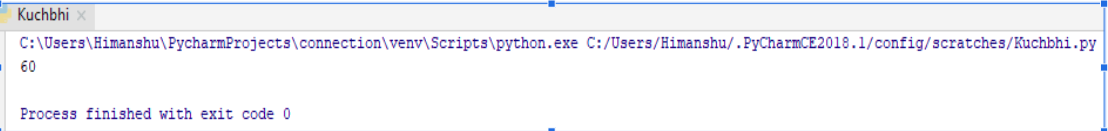So here it just return us a correct output.

### Use of Lambda function

We can use this function inside of any other anonymous function .

let's have an example :-

def myname (z):

return lambda a:a*z

mynam=myname(3)

print(mynam(11))

### Output of this Program

Here in above program first we created a function than through lambda function we tell to our Python interpreter that just multiply the argument which we passed on our ‘myname’ function , than we just make our ‘myname’ function equals to ‘mynam’ function. And pass the argument over there than through print method we just want to print our ‘mynam’ function to give us the output which must be multiplying with the argument which we had passed on our ‘myname’ function .
Let’s see what happens :-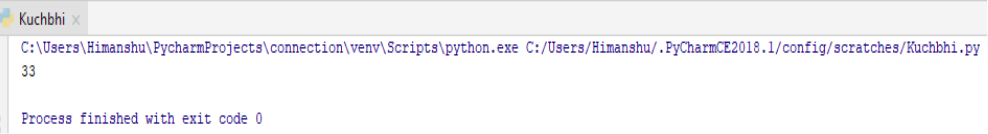So here we get the correct output.

We can also create as many function we want in the same above program
Let’s understand this by the following example :-

def myname (m):

return lambda x:x*m

mynam =myname (4)

myna =myname (5)

print (mynam(11))

print (myna(10))

### Output of this Program

Now here also we have created one function than pass lambda function over there to just multiply the arguments which we want to pass , than we created two different function makes them equals to our ‘myname’ function , than through print method we just want to print the value which we had passed in the form of arguments multiplied to the argument or value which we have passed over in our myname function .
Let's see what happens :-So here it just return us a correct output.

Lambda function are also used along with built- in function like filter(),map(),and reduce().

Use of lambda function with filter()

The filter() function use in python to take in function and list as a arguments. The function is called with all item with list a new list return contained items for function is true.
Let's understand this by an example in which we filter out the odd number in the list :-

my_list=[3,6,9,12,15,20,21,30,35,42]

new_list=list(filter(lambda x:(x%2!=0),my_list))

print(new_list)

### Output of this ProgramSo here it just filter out the odd numbers from the list

Use of lambda function with map()

The map function in python takes in a function and a list of argument.The function called lambda and the list and new list is returned which all contains all the modified items returns by the function each item.
Let's understand this by an example in which we double all the values in a list :-

my_list=[3,6,9,12,15,20,21,30,35,42]

new_list=list(map(lambda x:x*2,my_list))

print(new_list)

### Output of this Program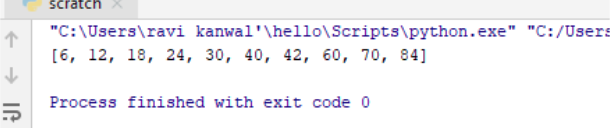So here it just doubles the value .

Use of lambda function with reduce()

The function called lambda function and the list and new reduce result in returned. This performs the repetitive operation over the pair of the list. This is part of functools module .
Let's understand this by an example

from functools import reduce # here we have import reduce function from functools module

my_fun = [1, 3, 5, 7, 9, 10]

multiply = reduce(lambda x, y: x * y), my_fun)

print (multiply)

### Output of this Program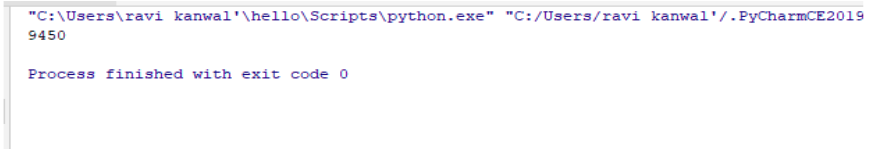So here we get the desired output.

python training insitute| Best IT Training classes in Gurgaon | python training